## 4.4.4 Energy Relaxation Times

The energy relaxation times are used in the HD mobility models, in the energy balance equations of the hydrodynamic transport model, and in the lattice heat flow equation. They can be either set constant or calculated using the following model depending on the carrier energy: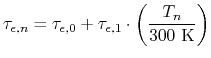(4.81)

For GaN and InN we use the parameter sets as listed in Table 4.12, while for AlN a constant relaxation time of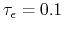ps is assumed. Special care is taken that the relaxation times never reach negative values even at high electric fields (for which Si models indeed yield negative erroneous results).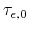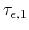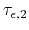GaN 0.21 0.004 0.0 InN 0.21 0.012 0.0

S. Vitanov: Simulation of High Electron Mobility Transistors